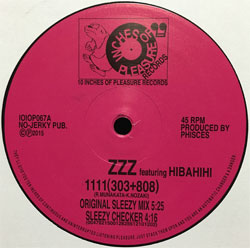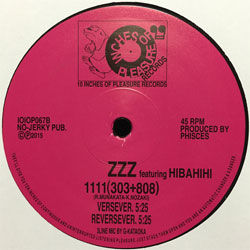# NLJNR20151226

#### NLJNR20151226

##### PLZ-JAH-PLZ-JAH

10″のご案内になります。

###### 10 INCHES OF PLEASUREIOIOP067AIOIOP067B

[IOIOP067] ZZZ featuring HIBAHIHI – 1111(303+808)

track listing
a1.Original Sleezy Mix 5’25″

a1.Sleezy Checker 4’16″

b1.Verse Ver. 5’25″

b1.Reverse Ver. 5’25″

###### HIP UP! HOUSE DOWN!

DJ Nozakiによるスリージー仕様のヒップでアッパーかつハウスでダウナーなビートに、元ブッダ・ブランドのNipps(エナイピーピーエス) aka
Hibahihi(飛葉飛火)がバースで修飾を施した今作は、MITグローバル・スタディーズ研究科文化人類学者ならびに日本のヒップホップ研究家
イアン・コンドリー教授をして「想像をZする」と言わしめた、10インチ史上最高の近未来フューチャー体験。

This 10″ sounds llike the Quiet Man meets a Tiny Girl in United Dairies.
Sleezy 808&303 Hip-Up and House-Down stylee featuring HIBAHIHI aka N.I.P.P.S.!

^v^v^v^v^v^v^v^v^v^v^v^v^v^v^v^v^v^v^v^v^v^v^v^v^v^v^v^v^v^v^v^v^v^v^v^v^v^v^v^v^v^v^v^v^v^v^v^v^v^v^v^v^v^v^v^v^v^v^v^v^v^v^v^v^v^v^v
DJ Nozaki’s collaboration with Nipps is like time travel.  I feel transported to the near future, where things are more trippy and more intense.
Minimalist, driving, hypnotic, and groove oriented – this is must-listen music for those interested in new sounds and new styles.  Nipps scores big
with his vocals, adding a hip-hop sensibility, which is deep – given his work with legendary rap group Buddha Brand – yet he brings a provocatively
new version of himself as well.  Nozaki takes us places we hadn’t imagined to go.  What fun to spend some time with this EP!
Text by Ian Condry, MIT Professor & author of Hip-Hop Japan (日本のヒップホップ)
^v^v^v^v^v^v^v^v^v^v^v^v^v^v^v^v^v^v^v^v^v^v^v^v^v^v^v^v^v^v^v^v^v^v^v^v^v^v^v^v^v^v^v^v^v^v^v^v^v^v^v^v^v^v^v^v^v^v^v^v^v^v^v^v^v^v^v

Presale:-JPY
Retail Price:Open